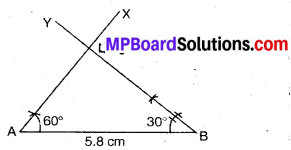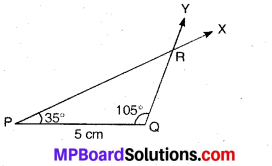# MP Board Class 7th Maths Solutions Chapter 10 Practical Geometry Ex 10.4

## MP Board Class 7th Maths Solutions Chapter 10 Practical Geometry Ex 10.4

Question 1.
Construct ∆ABC, given m ∠A = 60°, m ∠B = 30° and AB = 5.8 cm.
Solution:
Steps of Construction :1. Draw a line segment AB if 5.8 cm length.
2: Draw ∠BAX = 60°.
3. Draw ∠ABY = 30°.
4. Let AX and BY intersect at A.
Then, ABC is the required triangle.Question 2.
Construct ∆PQR if PQ = 5 cm, m ∠PQR = 105° m ∠QRP = 40°.
Solution:Here, ∠Q, ∠R and the side PQ are given. But to draw. We know that,
∠P + ∠Q + ∠R = 180° (∵ Sum of the angles of a triangle is 180°)
or ∠P + 105° + 40° = 180°
or ∠P = 180° – 145° = 35°
Thus, we have PQ = 5 cm,
∠P = 35° and ∠Q = 105°.
Steps of Construction :
1. Draw a line segment PQ if 5 cm length.
2: Draw ∠QPX = 35°.
3. Draw ∠PQY = 105°.
4. Let PX and QY intersect at R.
Then, PQR is the required triangle.

Question 3.
Examine whether you can construct ∆DEF such that EF = 7.2 cm, m ∠E = 110° and m ∠F = 80°. Justify your answer.
Solution:
Given, m∠E = 110° and m∠F = 80°
Since, m ∠E + m∠E = 110° + 80° = 190° > 180°
Thus, the ∆DEF cannot be drawn.